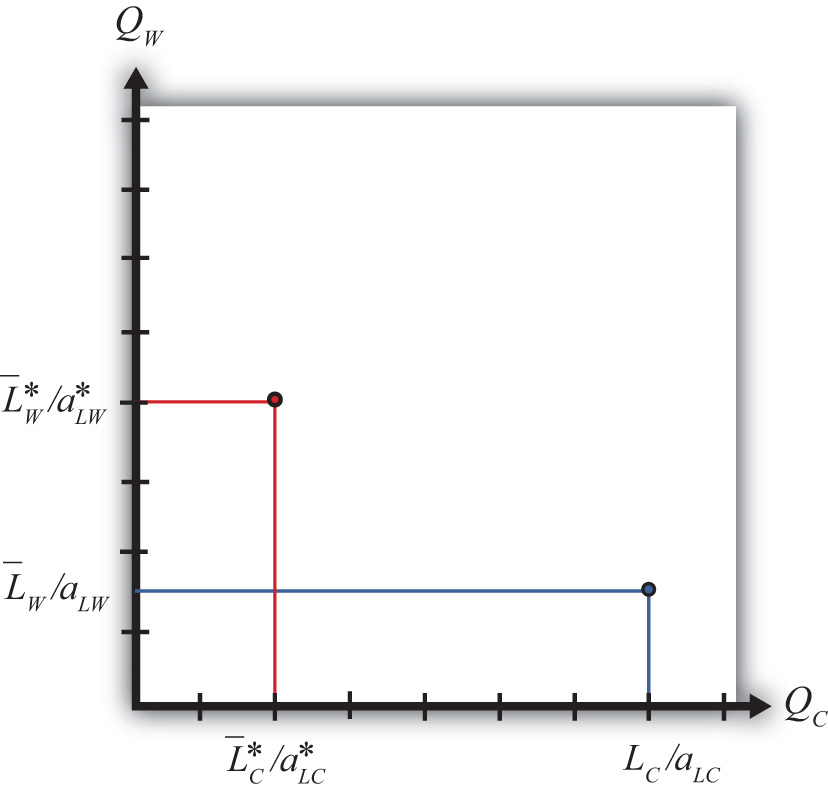This is “Autarky Equilibrium in the Immobile Factor Model”, section 4.6 from the book Policy and Theory of International Trade (v. 1.0). For details on it (including licensing), click here.

Has this book helped you? Consider passing it on:
Creative Commons supports free culture from music to education. Their licenses helped make this book available to you.
DonorsChoose.org helps people like you help teachers fund their classroom projects, from art supplies to books to calculators.

## 4.6 Autarky Equilibrium in the Immobile Factor Model

### Learning Objectives

1. Depict an autarky equilibrium in the immobile factor model.
2. Determine the autarky terms of trade given particular assumptions concerning technology, endowments, and demands.

Suppose two countries, the United States and France, have the exactly the same number of winemakers and cheesemakers. This means $L¯C=L¯C∗$ and $L¯W=L¯W∗$. Suppose also that the United States has an absolute advantage in the production of cheese, while France has the absolute advantage in the production of wine. This means $aLC and $aLW∗. Also, assume that the preferences for the two goods in both countries are identical.

For simplicity, let aggregate preferences be represented by a homothetic utility function. These functions have the property that for any price ratio, the ratio of the two goods consumed is equal to a constant. One function with this property is $QWDQCD=PCPW$, where $QCD$ is the aggregate quantity of cheese demanded and $QWD$ is the aggregate quantity of wine demanded. This function says that the ratio of the quantity of wine demanded to the quantity of cheese demanded must equal the price ratio.

For example, suppose that consumers face a price ratio PC/PW = 2 gallons of wine per pound of cheese. In this case, consumers will demand wine to cheese in the same ratio: two gallons per pound. Suppose the price ratio rises to PC/PW = 3. This means that cheese becomes more expensive than wine. At the higher price ratio, consumers will now demand three gallons of wine per pound of cheese. Thus as the relative price of cheese rises, the relative demand for wine rises as consumers substitute less expensive wine for more expensive cheese. Similarly, as the price of wine falls, the relative demand for wine rises.

The PPFs for the two countries in this case are plotted in Figure 4.2 "The U.S. and France’s PPFs". The United States produces more cheese than France, while France produces more wine than the United States. Because the factors are immobile, the ratio of wine to cheese production in the United States must be $QWQC=L¯W/aLWL¯C/aLC$.

Figure 4.2 The U.S. and France’s PPFsIn autarky, the quantity demanded of each good must equal the quantity supplied. This implies that the ratios of quantities must also be equalized such that $QWDQCD=QWQC$.

Substituting from above yields the autarky price ratio in the United States:

$( P C P W )Aut= L¯W/aLWL¯C/aLC = aLCaLWL¯WL¯C.$

Similarly, France’s autarky price ratio is the following:

$( P C ∗ P W ∗ )Aut= aLC∗aLW∗L¯W∗L¯C∗.$

Since by assumption the two countries have identical labor endowments, the United States has an absolute advantage in cheese production, and France has an absolute advantage in wine production, it follows that

$( P C P W )Aut< ( P C ∗ P W ∗ )Aut.$

Note that the same terms of trade relationship would follow if instead we assumed that the unit labor requirements, and hence the technologies, were the same in both countries but allowed the endowment of cheesemakers to be greater in the United States while the endowment of winemakers was larger in France.

In autarky, each country will produce at its production possibility point and, since there is no trade, will consume the same quantities of cheese and wine. The price of cheese is lower in the United States in autarky because it produces relatively more cheese than France given its absolute advantage, and that extra supply tends to force the price of cheese down relative to France. Similarly, France’s absolute advantage in wine causes it to produce more wine than the United States, which causes the price of wine in France to be lower than in the United States.

### Key Takeaways

• In autarky, in the immobile factor model, consumption will occur at the only production point possible in the model.
• The autarky terms of trade for a good will be lower in the country with the productivity advantage (or the greater factor endowment in that product).

### Exercise

1. Jeopardy Questions. As in the popular television game show, you are given an answer to a question and you must respond with the question. For example, if the answer is “a tax on imports,” then the correct question is “What is a tariff?”

1. This happens to the demand for cheese if the price ratio PC/PW rises.
2. This happens to the demand for cheese if one kilogram of cheese now trades for one liter of wine rather than two liters.
3. This happens to the demand for cheese if one liter of wine now trades for three kilograms of cheese rather than four kilograms.
4. With homothetic preferences, the ratio of consumer demands of wine to cheese will equal this other ratio.